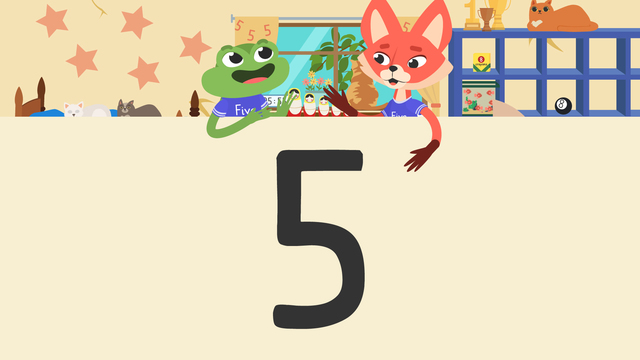# The Number 5Rating

Ø 4.5 / 10 ratings
The authorsTeam Digital

## The Number 5

In this text on the number 5 we will explore the appearance and the meaning of the number 5. We will learn how to spell the number 5, look at images of the number 5, and explore what the number 5 represents. Take a look below to learn all about the number 5.

## What Does the Number 5 Mean?

Let’s learn more about the number five and how to write it with the following explanation. When someone talks about the number five, they are talking about the number of things that you have. The number 5 meaning is that there are five items in a group! Let’s use the chart below to see where we find the number five. We start counting at the number 1.

1 one
2 two
3 three
4 four
5 five

## How to Write the Number 5

The number five has one line on top, one to the side and then a curve to the right. To write the number five, we need to start at the very top line, make one line across, then one line down, and finally a curve to the right! You can use this rhyme to help: Slide to the left, then slide down, make a curve nice and round!## The Number 5 – Summary

Today we learned all about the number five, how to write the number five, and groups of objects that represent the number five. So let’s repeat what the number five is in this summary.

How it looks 5
How it is spelled F I V E
How many it is ▲▲▲▲▲

Have you practiced yet? On this website, you can also find worksheets and exercises after the video so that you can say, “ I can show the number 5!”.

### TranscriptThe Number 5

Let's make room for ... "The Number Five." : "What's the number five anyway?" Today we will learn about the number five. When someone says there are five of something, they are talking about the number of objects. : "Like my FIVE stacking blocks? : "Or my FIVE piece nesting doll?" That's right, Skylar and Henry. Those are GREAT examples of the number FIVE. Can you see any other examples of groups of five in Henry and Skylar's room? There are FIVE cars on their bed sheets! Is there another example of the number FIVE? The number FIVE is all over their curtains! What does the number FIVE look like? (pause for think time) Skylar and Henry both have the number five on their shirts! The number five has two lines and a bump that curves to the right. How do you write the number five? To write the number five, we need to start at the very top line, make one line across, then one line down, and finally a curve to the right! There are different ways to remember how, let's hear a rhyme to help! Slide to the left, then slide down, make a curve nice and round! Can you help us to write the number five? Let's write the number five! Every time you hear the word 'slide' slide in that direction. Slide to the left, then slide down, make a curve nice and round! One more time! Slide to the left, then slide down, make a curve nice and round! Now THAT is a great looking five, thanks for helping! Today we learned all about the number FIVE. We learned about objects in sets of five, the number five, and how to write it! Up for a challenge? Watch this video again and see if you can find the FIVE stars, FIVE flowers, and FIVE cats hidden in the playroom! Comment below when you find them! If you want to learn more, check out the next video on the number SIX.